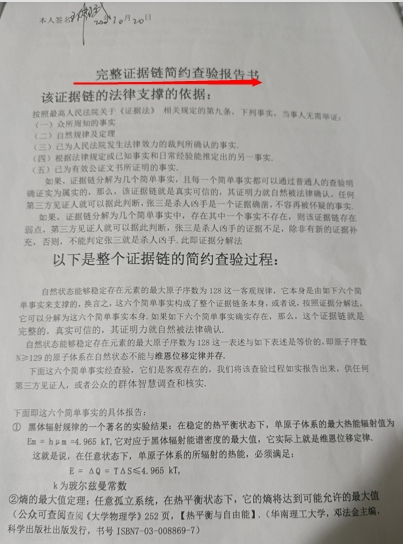--------“自然界能够稳定存在的元素的最大原子序数为128的客观自然规律的首次发现权”的申报人的离奇经历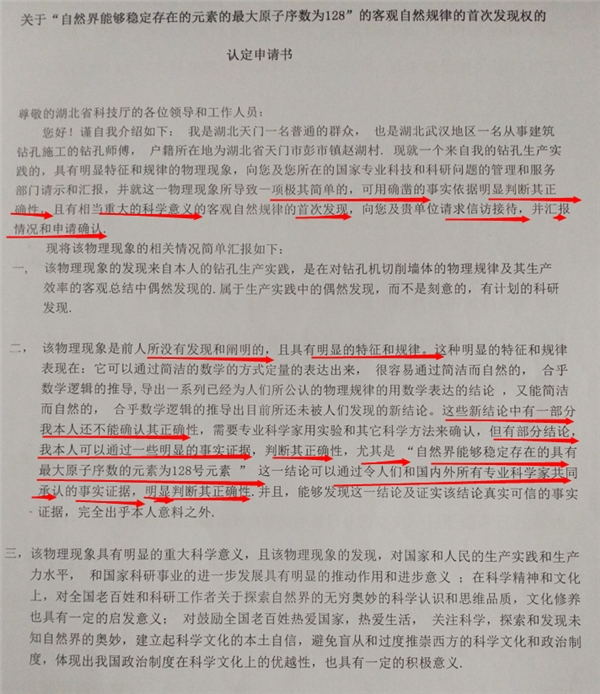1. 从平凡的发现到非凡的发现

E =∮F0dL=ε∂H0/∂t=∬ (▽×F0) ·dS=∬ (m/π)ε(∂W0/∂t)· dS

∬m(▽×W0) dS =-ηd(∬F0· dS )/ dt =-η∬(∂F0/∂t)·dS -η∬(▽·F0)V ·dS

▽×a = (1/π)ε(∂W0/∂t)  ①

▽×W0 = - η∂a /∂t-ηj   ②

a = W0×V为体系的加速度.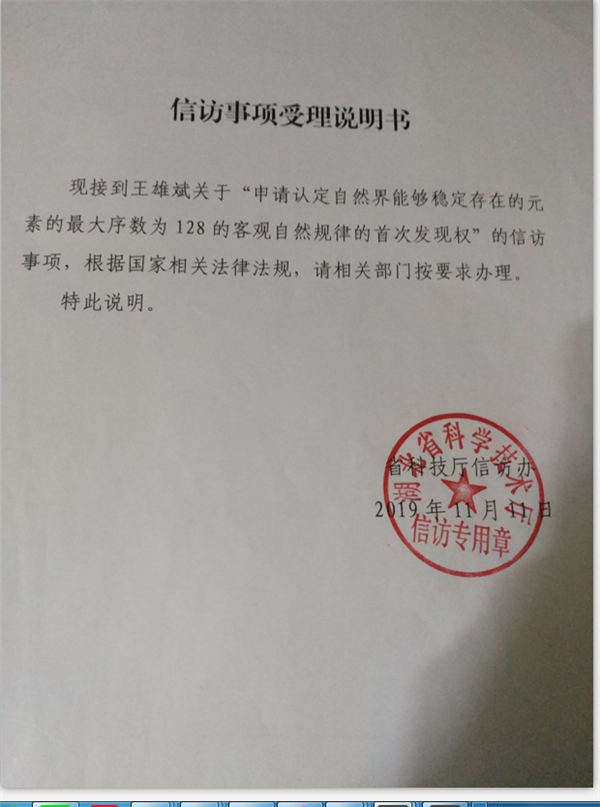▽×a = - 2∂W/∂t  ③

▽×W = (1/2C^2)∂a /∂t-q^2ρV/ε0mC^2 ④

a = W×V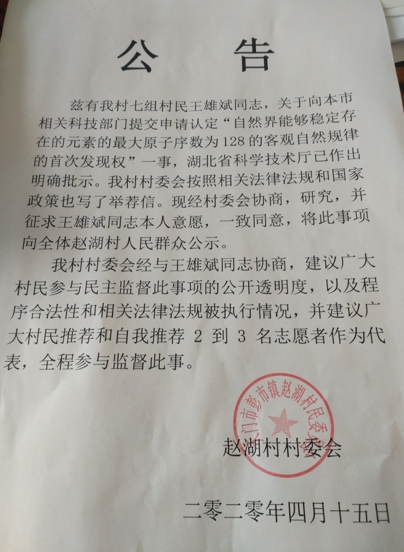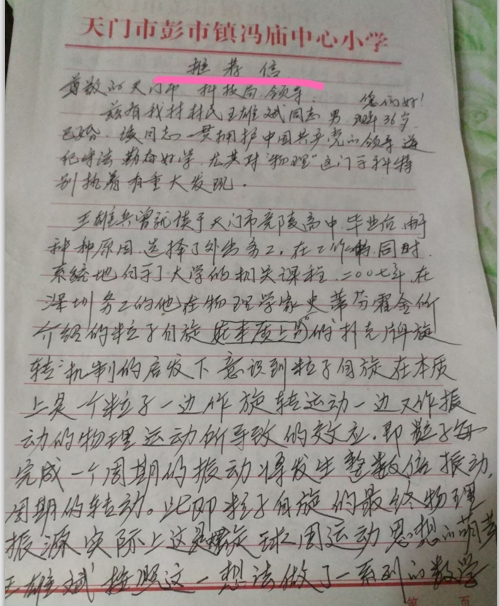2 .从平凡的方程到非凡的结论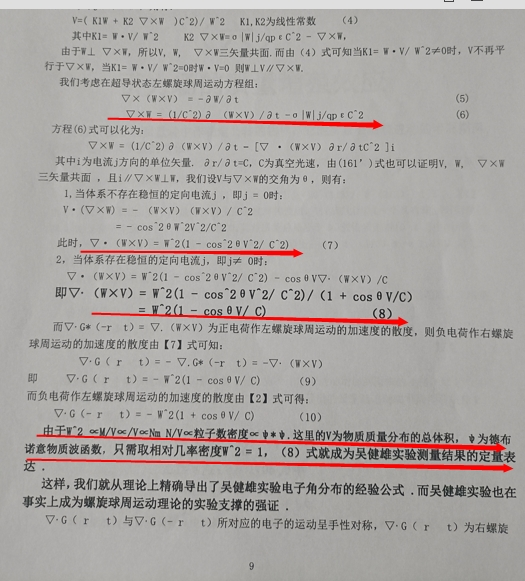θaº = 12×180Z^2·e^2·( 1 + 1 /ζ) /( n^2 ·a·4πεmC^2)≈1.1º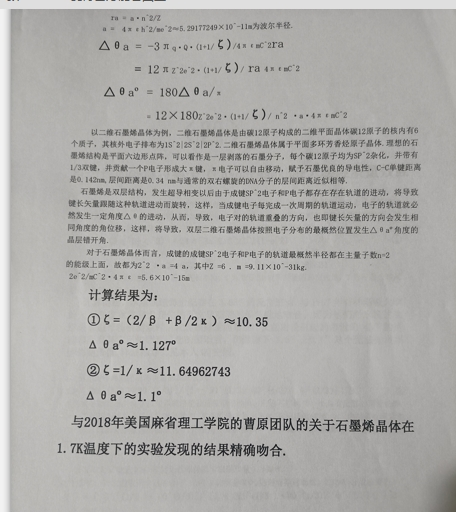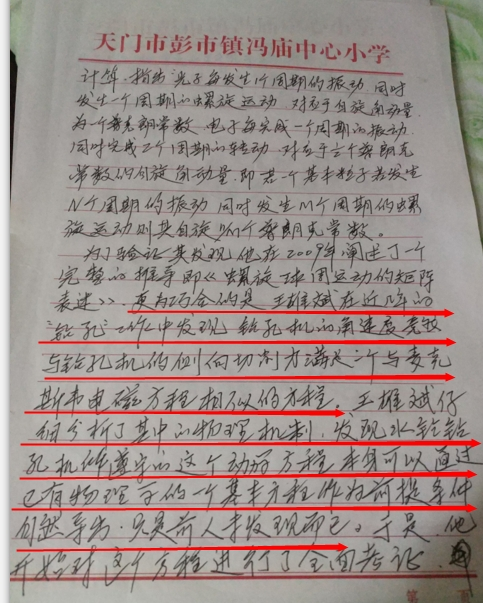3 .从钻孔机到元素周期表的最后一号元素.

1.按照方程组③④的一般形式对螺旋星系轨道的精确求解:在任意无旋力场的施瓦西茲半径处将普遍存在如下轨道方程: R = Rs.e^βθ +ζθ

2.他利用了广义相对论的一项经典结论:即任意粒子和物体在一个力场的施瓦西半径附近时,它将自然地发生3π的进动.

3.他利用了量纲分析的一个结果.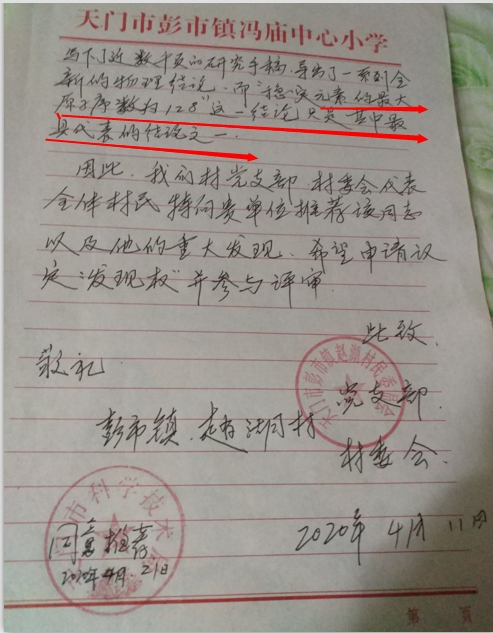C0max = Q max·m^3/C •s^2 = 4πG Mmax = 128.665911·q ·m^3/C •s^2

≈128(2/3)q ·m^3/C •s^2

E[128(1/3)]E(128)的计算结果如下: